# Paper Folding UPSC Questions

## Paper Folding MCQ Questions

1.

Find the image which would mostly matches with the folded form given below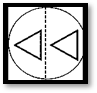A.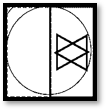B.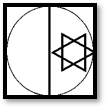C.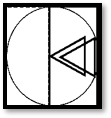D.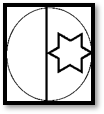A.2.

How many dots lie opposite to the face having three dots, when the given figure is folded to form a cube?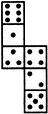A.

2

B.

4

C.

6

D.

8

C. 6

3.

Find the image which would mostly matches with the unfolded form given below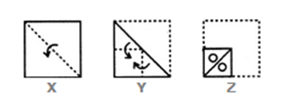A.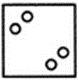B.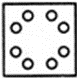C.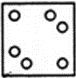D.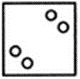B.4.

Find the image which would mostly matches with the unfolded form given below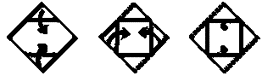A.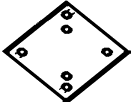B.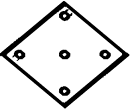C.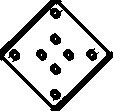D.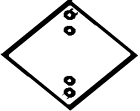D.5.

In a figure a transparent sheet is given. Find out the pattern formed when the transparent sheet is folded . (Dotted line represent the folding of paper)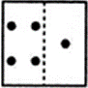A.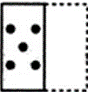B.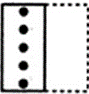C.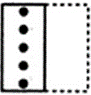D.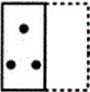A.6.

In a figure a transparent sheet is given. Find out the pattern formed when the transparent sheet is folded . (Dotted line represent the folding of paper)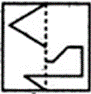A.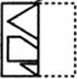B.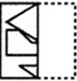C.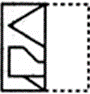D.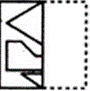C.